Hostname: page-component-59f8fd8595-hk2jd Total loading time: 0 Render date: 2023-03-22T01:08:22.330Z Has data issue: true Feature Flags: { "useRatesEcommerce": false } hasContentIssue true

# MODEL-THEORETIC PROPERTIES OF ULTRAFILTERS BUILT BY INDEPENDENT FAMILIES OF FUNCTIONS

Published online by Cambridge University Press:  17 April 2014

## Abstract

Our results in this paper increase the model-theoretic precision of a widely used method for building ultrafilters, and so advance the general problem of constructing ultrafilters whose ultrapowers have a precise degree of saturation. We begin by showing that any flexible regular ultrafilter makes the product of an unbounded sequence of finite cardinals large, thus saturating any stable theory. We then prove directly that a “bottleneck” in the inductive construction of a regular ultrafilter on λ (i.e., a point after which all antichains of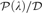${\cal P}\left( \lambda \right)/{\cal D}$ have cardinality less than λ) essentially prevents any subsequent ultrafilter from being flexible, thus from saturating any nonlow theory. The constructions are as follows. First, we construct a regular filter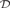${\cal D}$ on λ so that any ultrafilter extending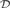${\cal D}$ fails to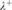${\lambda ^ + }$-saturate ultrapowers of the random graph, thus of any unstable theory. The proof constructs the omitted random graph type directly. Second, assuming existence of a measurable cardinal κ, we construct a regular ultrafilter on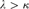$\lambda > \kappa$ which is λ-flexible but not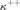${\kappa ^{ + + }}$-good, improving our previous answer to a question raised in Dow (1985). Third, assuming a weakly compact cardinal κ, we construct an ultrafilter to show that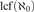${\rm{lcf}}\left( {{\aleph _0}} \right)$ may be small while all symmetric cuts of cofinality κ are realized. Thus certain families of precuts may be realized while still failing to saturate any unstable theory.

Type
Articles
Information
The Journal of Symbolic Logic , March 2014 , pp. 103 - 134

## Access options

Get access to the full version of this content by using one of the access options below. (Log in options will check for institutional or personal access. Content may require purchase if you do not have access.)

## References

#### REFERENCES

Chang, C. C. and Keisler, H. J., Model theory, North-Holland, New York, 1973.Google Scholar
Dow, A., Good and OK ultrafilters. Transactions of the American Mathematical Society, vol. 290 (1985), no. 1, pp. 145160.CrossRef
Džamonja, M. and Shelah, S., On ◁* -maximality. Annals of Pure and Applied Logic, vol. 125 (2004), pp. 119158.CrossRef
Erdös, E., Hajnal, A., Mate, A., and Rado, R.. Combinatorial set theory: Partition relations for cardinals, Studies in Logic and the Foundations of Mathematics, vol. 106. North-Holland, Amsterdam, 1984.Google Scholar
Engelking, R. and Karłowicz, M., Some theorems of set theory and their topological consequences. Fundamenta Mathematicae, vol. 57 (1965), pp. 275285.
Kanamori, A., The higher infinite, Springer, Berlin, 2003.Google Scholar
Keisler, H. J., Good ideals in fields of sets.Annals of Mathematics, vol. 79 (1964), no. 2,pp. 338359.
Keisler, H. J., Ultraproducts which are not saturated, this Journal, vol. 32 (1967), pp. 2346.
Kennedy, J., Shelah, S., and Vaananen, J., Regular ultrafilters and finite square principles, this Journal, vol. 73 (2008), pp. 817823.
Kunen, K., Ultrafilters and independent sets. Transactions of the American Mathematical Society, vol. 172 (1972), pp. 299306.CrossRef
Malliaris, M., Realization of φ-types and Keisler’s order. Annals of Pure and Applied Logic, vol. 157 (2009), no. 2–3, pp. 220224.CrossRef
Malliaris, M., Ph. D. thesis, U.C. Berkeley (2009), available at http://math.uchicago.edu/∼mem.
Malliaris, M., Hypergraph sequences as a tool for saturation of ultrapowers. this Journal, vol. 77 (2012), no. 1, pp. 195223.
Malliaris, M., Independence, order and the interaction of ultrafilters and theories. Annals of Pure and Applied Logic, vol. 163 (2012), no. 11, pp. 15801595.
Malliaris, M. and Shelah, S., Constructing regular ultrafilters from a model-theoretic point of view. Transactions of the American Mathematical Society, available at http://www.ams.org/cgi-bin/mstrack/accepted_papers?jrnl=tran.
Malliaris, M. and Shelah, S., A dividing line within simple unstable theories. Advances in Mathematics, vol. 249 (2013).
Malliaris, M. and Shelah, S., Cofinality spectrum theorems in model theory, set theory and general topology. math.LO/1208.5424.
Shelah, S., Classification theory, North-Holland, Amsterdam, 1978.Google Scholar
Shelah, S., Classification theory and the number of non-isomorphic models, revised edition, North-Holland, Amsterdam, 1990.Google Scholar
Shelah, S., Toward classifying unstable theories. Annals of Pure and Applied Logic, vol. 80 (1996), pp. 229255.CrossRef